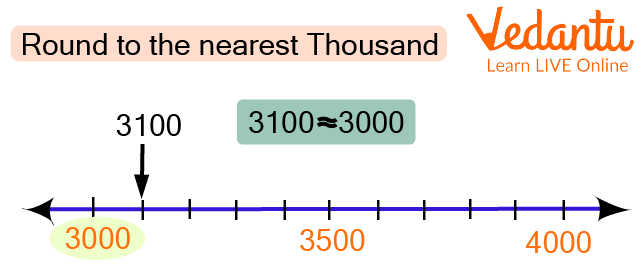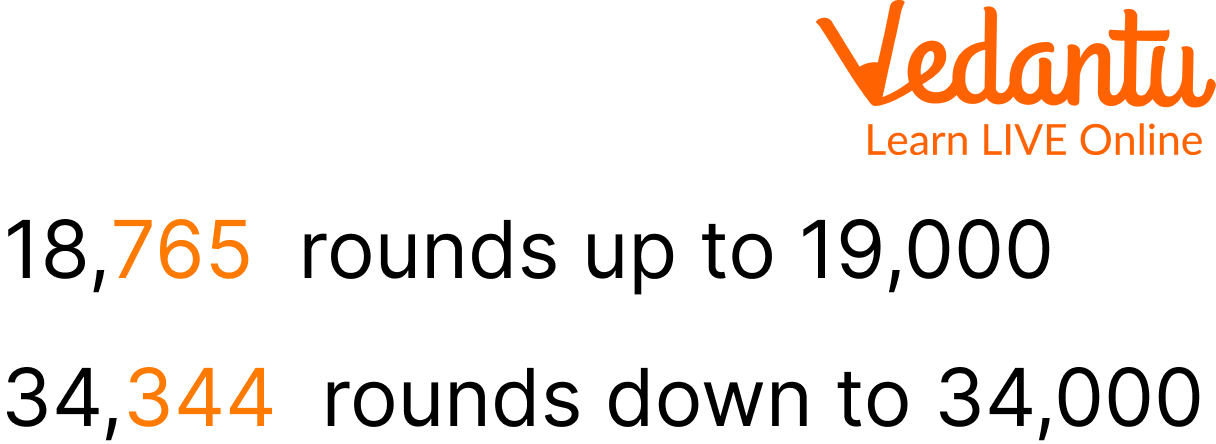Courses
Courses for Kids
Free study material
Free LIVE classes
More

# Rounding Off to The Nearest 1000LIVE
Join Vedantu’s FREE Mastercalss

## What is Rounding Off of a Number?

Rounding off numbers is an interesting concept. It rounds off a number to the nearest tens, hundreds, thousands, or so on. This concept is used in our daily life. For example, there are 495 students in a school, so we round off the number and say that there are around 500 students in the school. Let us understand the full concept of what is the nearest thousand and nearest hundred and Rounding off to the Nearest 1000 and 100 with a few rounds to the nearest thousand examples.

## Number Line Representation

Let us understand the concept of rounding to the nearest thousand examples through number line representation.Round to Nearest Thousand

In this example, we can understand that below 3500 numbers would round off to 3000, and above 3500 would round off to 4000. So 3450 would round off to 3000 and 3890 would round off to 4000. This will be followed in all the numbers which need to get rounded off to the nearest thousand.

## Rounding Numbers to The Nearest 1000

To round to the nearest thousand examples we write the estimated value which is a simpler representation of the number.Round to Nearest Thousand

We have to understand the place value of the numbers in rounding off to the nearest concept. To round off to the nearest thousand, we need to write the multiple of 1000 which is closest to that specific number. For example, 1120 students are studying in a university, in this case, we usually say that there are around 1000 students in round figures.

## Solved Examples

Q 1. Round 3240 to the Nearest thousand

Ans: To solve this question we have to see whether 3240 is closer to 3000 or 4000 in the counting series. In this case, 3240 is closer to 3000, hence the round-off figure of 3240 is 3000.

Q 2. Round 7980 to the Nearest thousand

Ans: To solve this question we have to check whether 7980 is closer to 7000 or 8000 in the counting. In this case, 7980 is closer to 8000, hence 8000 is the round figure of 7980.

Q 3. Round 8039 to the Nearest Thousand

Ans: To solve this question we have to check whether 8039 is closer to 8000 or 9000 in the counting. In this case, 8039 is closer to 8000, hence 8000 is the round figure of 8039.

Q 4. Round 16796 to the Nearest Thousand

Ans: To solve this question we have to check whether 16796 is closer to 16000 or 17000 in the counting. In this case, 16796 is closer to 16000, hence 17000 is the round figure of16796.

## Practice Questions

Q 1. What is the nearest thousand of 5321?

Ans: 5000 is the round-off number.

Q 2. What is the nearest thousand of 8999?

Ans: 9000 is the nearest thousand.

Q 3. What is the nearest thousand of 6294?

Ans: 6000 is the nearest thousand.

Q 4. What is the nearest thousand 1964?

Ans: 2000 is the nearest thousand.

Q 5. What is the nearest thousand of 9839

Ans: 10000 is the nearest thousand.

## Summary

Rounding Numbers to the nearest thousand is a similar concept to rounding numbers to the nearest tens or hundred. We use this concept in our daily lives while counting money or buying things. Whenever we go to the market for shopping, we keep money with us in round figures. Rounding off the numbers to the nearest tens, hundred, and thousand is done by keeping the place value of digits in mind. Kids must learn and understand this topic in greater detail as it helps in various other concepts and real life.

Last updated date: 26th Sep 2023
Total views: 112.5k
Views today: 1.12k

## FAQs on Rounding Off to The Nearest 1000

1. Why do we round off?

Rounding numbers make them simpler and easier to use. Although they're slightly less accurate, their values are still relatively close to what they originally were. People round numbers in many different situations, including many real-world situations you'll find yourself in on a regular basis.

2. Is 5 rounded up or down?

If the digit is less than 5, round the previous digit down; if it's 5 or greater, round the previous digit up.

3. Which numbers cannot be rounded off?

0 and negative numbers cannot be rounded off.